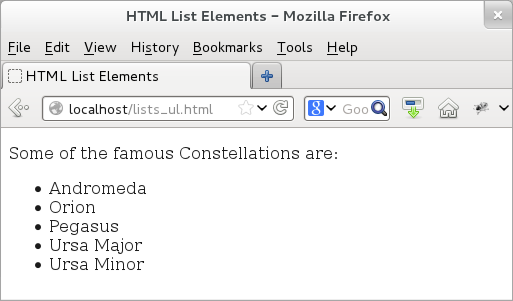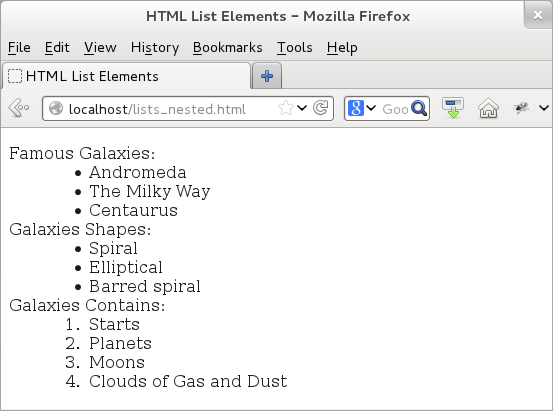## CodingBison

Sometimes the best way to present a set of data is to present them as a list. Luckily, HTML provides three different elements to do that! These elements are: ordered lists (identified by <ol> tag), unordered lists (identified by <ul> tag), and definition lists (identified by <dl> tag).

An ordered list displays numbers while an unordered list displays bullets. When we use an ordered list (<ol> element), then the key is emphasis on the order. Thus, if we have an example list, where we would like to work on item number 1 first, followed by item number 2, and so on, then using the ordered list is the way to go. If ordering is not important, then using an unordered list would be the way to go.

The tags for these list-types enclose the entire list of elements. To display individual items in the list, HTML provides yet another element: the list element that is identified by the <li> tag. W3C specification says that for the list element (<li>), the end tag (</li>) is optional.

The definition list (identified by <dl> tag) does not use the <li> element. Instead, it uses <dt> for data term and <dd> for data definition. Thus, to represent one term/definition pair, we would need a pair of <dt> and <dd> element.

### Examples

We begin with an example of an ordered list. The example (given below) provides a list of inner planets of our Solar System. Here the ordering is important since we start by listing the planet closest to the sun, then the next, and so on. You might note that we keep the closing tag for the <li> element but as per the specification, it is optional -- so take your pick!

``` <!doctype html>
<html>
<title>HTML List Elements</title>
<body>
<p> The inner planets of our Solar System are: </p>
<ol>
<li>Mercury </li>
<li>Venus </li>
<li>Earth </li>
<li>Mars </li>
</ol>
</body>
</html>
```

If we were to load the above page, then this time we would see the output displaying an ordered list.Figure: HTML Ordered List

Let us now rewrite another example that also has a list of items but this time, the ordering is not so important. Accordingly, we use an unordered list.

``` <!doctype html>
<html>
<title>HTML List Elements</title>
<body>
<p> Some of the famous Constellations are: </p>
<ul>
<li>Andromeda </li>
<li>Orion </li>
<li>Pegasus </li>
<li>Ursa Major </li>
<li>Ursa Minor </li>
</ul>
</body>
</html>
```

If we were to load the above page, then this time we would see the output as an unordered list.Figure: HTML Unordered List

Now that we have seen examples of both ordered lists and unordered lists, let us see an example of definition list. Here we present list-based content such that the ordering is not important and also the data is in the term/definition format. Like the case of <li> elements, the closing tag for <dt> and <dd> are optional. If you feel cozier with the closing tags, feel free to add them!

``` <!doctype html>
<html>
<title>HTML List Elements</title>
<body>
<p> Categorization of planets in our Solar System </p>

<dl>
<dt>Inner Planets: </dt>
<dd>The 4 innermost planets: Mercury, Venus, Earth, and Mars.</dd>
<dt>Outer Planets </dt>
<dd>The 4 outermost planets: Jupiter, Saturn, Uranus, and Neptune.</dd>
<dt>Dwarf Planets</dt>
<dd>Smaller planets at the edge of Solar System: Pluto, Makemake, Eris.</dd>
</dl>
</body>
</html>
```Figure: HTML Definition List

Our earlier examples focused on content such that they could be displayed using one list. However, it is possible that we can have data such that each element could be better represented by yet another list. For such cases, we can have nested lists. It is perfectly okay to nest the above three different types of list structure within one another. The following example demonstrates this structure, where we have a definition list such that each <dd> element of the definition list holds a (child) list element.

``` <!doctype html>
<html>
<title>HTML List Elements</title>
<body>
<dl>
<dt>Famous Galaxies: </dt>
<dd>
<ul>
<li> Andromeda </li>
<li> The Milky Way </li>
<li> Centaurus </li>
</ul>
</dd>

<dt>Galaxies Shapes: </dt>
<dd>
<ul>
<li> Spiral </li>
<li> Elliptical </li>
<li> Barred spiral </li>
</ul>
</dd>

<dt>Galaxies Contains: </dt>
<dd>
<ol>
<li> Stars </li>
<li> Planets </li>
<li> Moons </li>
<li> Clouds of Gas and Dust </li>
</ol>
</dd>
</dl>
</body>
</html>
```

The output of this example (given below) has top level terms followed by their definitions. The only difference is that definition themselves are also lists.Figure: HTML Nested Lists